# Don’t Solve the Normal Equations

The (ordinary) linear least squares problem is as follows: given an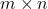matrix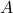and a vector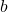of length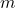, find the vector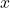such that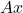is as close toas possible, when measured using the two-norm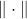. That is, we seek to

(1)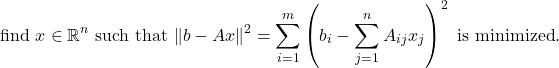From this equation, the name “least squares” is self-explanatory: we seekwhich minimizes the sum of the squared discrepancies between the entries ofand.

The least squares problem is ubiquitous in science, engineering, mathematics, and statistics. If we think of each row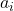ofas an input and its corresponding entry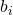ofas an output, then the solutionto the least squares model gives the coefficients of a linear model for the input–output relationship. Given a new previously unseen input, our model predicts the output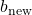is approximately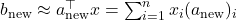. The vectorconsists of coefficients for this linear model. The least squares solution satisfies the property that the average squared difference between the outputand the prediction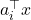is as small as it could possibly be for all choices of coefficient vectors.

How do we solve the least squares problem? A classical solution approach, ubiquitous in textbooks, is to solve a system of linear equations known as the normal equations. The normal equations associated with the least squares problem (1) are given by

(2)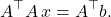This system of equations always has a solution. Ifhas full column-rank, then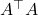is invertible and the unique least squares solution to (1) is given by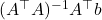. We assume thathas full column-rankQ for the rest of this discussion. To solve the normal equations in software, we computeand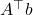and solve (2) using a linear solver like MATLAB’s “\”.1Even better, we could us a Cholesky decomposition since the matrixis positive definite. (As is generally true in matrix computations, it is almost never a good idea to explicitly form the inverse of the matrix, or indeed any matrix.) We also can solve the normal equations using an iterative method like (preconditioned) conjugate gradient.

The purpose of the article is to advocate against the use of the normal equations for solving the least squares problems, at least in most cases. So what’s wrong with the normal equations? The problem is not that the normal equations aren’t mathematically correct. Instead, the problem is that the normal equations often lead to poor accuracy for the least squares solution using computer arithmetic.

Most of the time when using computers, we store real numbers as floating point numbers.2One can represent rational numbers on a computer as fractions of integers and operations can be done exactly. However, this is prone to gross inefficiencies as the number of digits in the rational numbers can grow to be very large, making the storage and time to solve linear algebra problems with rationals dramatically more expensive. For these reasons, the vast majority of numerical computations use floating point numbers which store only a finite number of digits for any given real number. In this model, except for extremely rare circumstances, rounding errors during arithmetic operations are a fact of life. At a coarse level, the right model to have in your head is that real numbers on a computer are stored in scientific notation with only 16 decimal digits after the decimal point.3This is a simplification in multiple ways. First, computers store numbers in binary and thus, rather than storing 16 decimal digits after the decimal point, they store 52 binary digits. This amounts to roughly 16 decimal digits. Secondly, there are different formats for storing real numbers as floating point on a computer with different amounts of stored digits. The widely used IEEE double precision format has about 16 decimal digits of accuracy; the IEEE single precision format has roughly 8. When two numbers are added, subtracted, multiplied, and divided, the answer is computed and then rounded to 16 decimal digits; any extra digits of information are thrown away. Thus, the result of our arithmetic on a computer is the true answer to the arithmetic problem plus a small rounding error. These rounding errors are small individually, but solving an even modestly sized linear algebra problem requires thousands of such operations. Making sure many small errors don’t pile up into a big error is part of the subtle art of numerical computation.

To make a gross simplification, if one solves a system of linear equations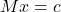on a computer using a well-designed piece of software, one obtains an approximate solution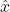which is, after accounting for the accumulation of rounding errors, close to. But just how close the computed solutionand the true solutionare depends on how “nice” the matrix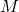is. The “niceness” of a matrixis quantified by a quantity known as the condition number of, which we denote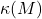.4In fact, there are multiple definitions of the condition number depending on the norm which one uses the measure the sizes of vectors. Since we use the 2-norm, the appropriate 2-norm condition numberis the ratio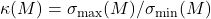of the largest and smallest singular values of. As a rough rule of thumb, the relative error betweenandis roughly bounded as

(3)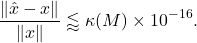The “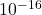corresponds to the fact we have roughly 16 decimal digits of accuracy in double precision floating point arithmetic. Thus, if the condition number ofis roughly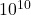, then we should expect around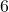digits of accuracy in our computed solution.

The accuracy of the least squares problem is governed by its own condition number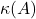. We would hope that we can solve the least squares problem with an accuracy like the rule-of-thumb error bound (3) we had for linear systems of equations, namely a bound like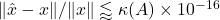. But this is not the kind of accuracy we get for the least squares problem when we solve it using the normal equations. Instead, we get accuracy like

(4)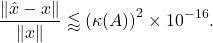By solving the normal equations we effectively square the condition number! Perhaps this is not surprising as the normal equations also more-or-less square the matrixby computing. This squared condition number drastically effects the accuracy of the computed solution. If the condition number ofis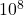, then the normal equations give us absolute nonsense for; we expect to get no digits of the answercorrect. Contrast this to above, where we were able to getcorrect digits in the solution todespite the condition number ofbeing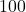times larger than!

All of this would be just a sad fact of life for the least squares problem if the normal equations and their poor accuracy properties were the best we could do for the least squares problem. But we can do better! One can solve linear least squares problems by computing a so-called QR factorization of the matrix.5In MATLAB, the least squares problem can be solved with QR factorization by calling “A\b”. Without going into details, the upshot is that the least squares solution by a well-designed6One way of computing the QR factorization is by Gram–Schmidt orthogonalization, but the accuracy properties of this are poor too. A gold-standard way of computing the QR factorization by means of Householder reflectors, which has excellent accuracy properties. QR factorization requires a similar amount of time to solving the normal equations and has dramatically improved accuracy properties, achieving the desirable rule-of-thumb behavior7More precisely, the rule of thumb is like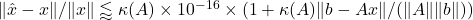. So even if we solve the least squares problem with QR factorization, we still get a squared condition number in our error bound, but this condition number squared is multiplied by the residual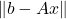, which is small if the least squares fit is good. The least squares solution is usually only interesting when the residual is small, thus justifying dropping it in the rule of thumb.

(5)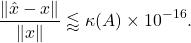I have not described how the QR factorization is accurately computed nor how to use the QR factorization to solve least squares problems nor even what the QR factorization is. All of these topics are explained excellently by the standard textbooks in this area, as well as by publicly available resources like Wikipedia. There’s much more that can be said about the many benefits of solving the least squares problem with the QR factorization,8E.g., it can work for sparse matrices while the normal equations often do not, it has superior accuracy to Gaussian elimination with partial pivoting even for solving linear systems, the “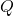” matrix in the QR factorization can be represented implicitly as a product of easy-to-compute-with Householder reflectors which is much more efficient when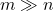, etc. but in the interest of brevity let me just say this: TL;DR when presented in the wild with a least squares problem, the solution method one should default to is one based on a well-implemented QR factorization, not solving the normal equations.

Suppose for whatever reason we don’t have a high quality QR factorization algorithm at our disposal. Must we then resort to the normal equations? Even in this case, there is a way we can reduce the problem of solving a least squares problems to a linear system of equations without squaring the condition number! (For those interested, to do this, we recognize the normal equations as a Schur complement of a somewhat larger system of linear equations and then solve that. See Eq. (7) in this post for more discussion of this approach.)

The title of this post Don’t Solve the Normal Equations is deliberately overstated. There are times when solving the normal equations is appropriate. Ifis well-conditioned with a small condition number, squaring the condition number might not be that bad. If the matrixis too large to store in memory, one might want to solve the least squares problem using the normal equations and the conjugate gradient method.

However, the dramatically reduced accuracy of solving the normal equations should disqualify the approach from being the de-facto way of solving least squares problems. Unless you have good reason to think otherwise, when you see, solve a different way.